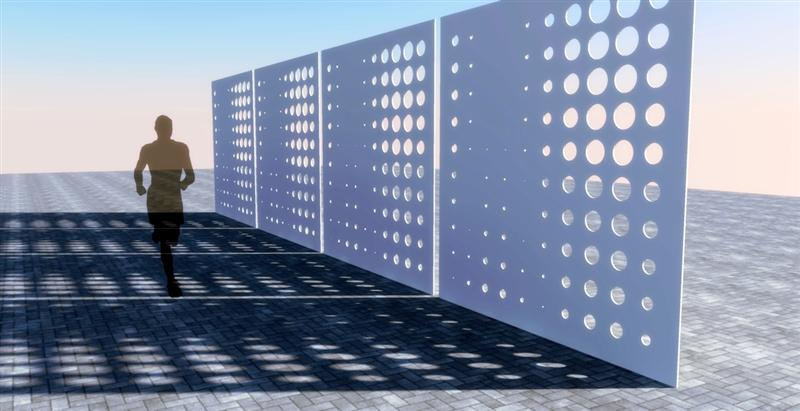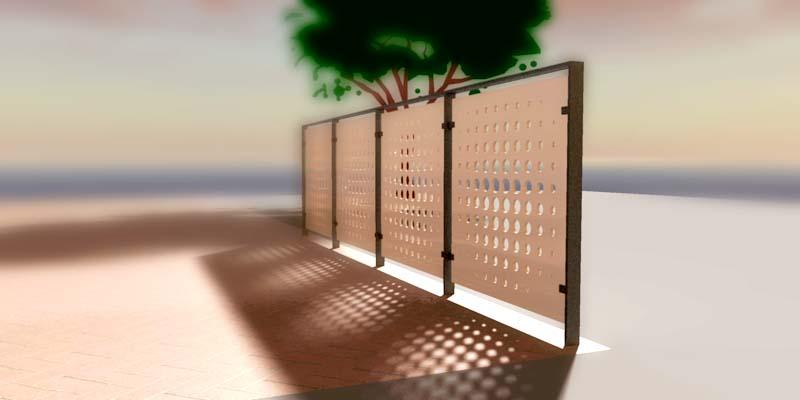# SketchUp script clips #2: Creating geometryIn this section, we’ll start creating scripted geometry in SketchUp. In this and all following posts, I’ll approach SketchUp scripting in a pragmatic way. This means that I don’t think every step in the process should be scripted – after all, I am not writing a plugin. I’ll only script what can’t efficiently be modeled by hand and I’ll use standard SketchUp modeling tools to finish up a project.

By the way: Check out my book, “Architectural Design with SketchUp: 3D Modeling, Extensions, BIM, Rendering, Making, and Scripting.” In chapter 7, I describe scripting in SketchUp in more detail.

Before you get going, make sure you have read the first installment of this series and have the latest version of the Ruby Code Editor installed.

Creating geometry is pretty easy with the SketchUp Ruby API. In essence, you will be adding to the collection of entities in the current model. To do this, you can use any of these methods of the entities object:

Entities (Parent: Object)
.at .clear! .count .each .erase_entities
.fill_from_mesh .intersect_with .length
.model .parent .remove_observer
.transform_by_vectors
.transform_entities

Once you get a hold of the entities collection using the following line, simply add to the collection whatever you need.

`entities = Sketchup.active_model.entities`

To get us started, let’s create a 3D grid of regularly spaced boxes. In the following little bit of code, we’ll define a number of boxes per axis (“n”), a box spacing (“s”) and a box width (“w”). Please note: All lengths are entered as numbers, which means that they default to inches. If you need to use other units, write dimensions like this:

```s = "1m".to_l
```

Paste the following code into the Ruby Code Editor in SketchUp and click on “Run Code”:

```# Creates a 3D grid of boxes

entities = Sketchup.active_model.entities

n = 5
s = 100
w = 20

(0..n-1).each { |i|
(0..n-1).each { |j|
(0..n-1).each { |k|
face.pushpull -w
}
}
}```

This will be the result:While this gets the job done, it is not very efficient. After all, we are creating geometry for each of the many boxes. In SketchUp, the approach that takes less computational effort and less space on the harddisk is usually to create one box, convert it to a component and then just insert component instances. Instead of saving a whole lot of geometry, only insertion points are then being saved. The following code does this by creating a grouped box, converting it into a component and then copying it multiple times using transformations.

```# Creates a 3D grid of boxes using components

n = 5
s = 100
w = 20

entities = Sketchup.active_model.entities
face.pushpull -w
comp = group.to_component

(0..n).each { |i|
(0..n).each { |j|
(0..n).each { |k|
transformation = Geom::Transformation.new([i*s,j*s,k*s])
}
}
}```

I’ll cover transformations (move, copy, rotate, scale) in detail in a later post.

Scripting geometry becomes especially effective when we use a non-linear formula to define size, position etc. The next piece of code creates a rectangular grid of circles whose size is determined in two directions by a sine function.

Paste the following code into the Ruby Code Editor in SketchUp and click on “Run Code”:

```# Creates a square panel with a sinusoidal hole pattern

entities = Sketchup.active_model.entities

width = 36
n = 10
s = width/(n+1).to_f

(0..n-1).each { |i|
(0..n-1).each { |j|
}
}```

Here’s the resulting panel after using the pushpull tool:You can get creative with this little bit of code if you replace some of the “sin” functions with “cos” and also play with the factors that end up determining circle diameter (the last parameter in the “add_circle” method). A variation of this is shown in the topmost image in this post.

One thing that stumped me at first was Ruby’s reluctance to give me a floating point value after dividing two integers. That is the reason why you see “.to_f” in a few places in the code. This little snippet converts a number to a “float”. In addition, it may be possible that you need to add the Math class to the “sin” function. It works fine for me as shown but the long version would be:

```Math.sin(x)
```

By the way: The best way to access the reference for the “add_circle” method while you are coding is of course the Reference Browser tab directly within the Ruby Code Editor:Happy scripting!

Last in this series: Setup and basic ideas
Next in this series: More to follow soon…